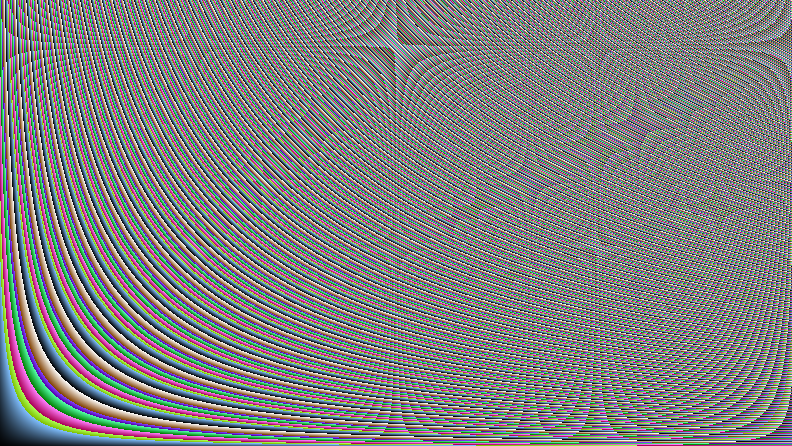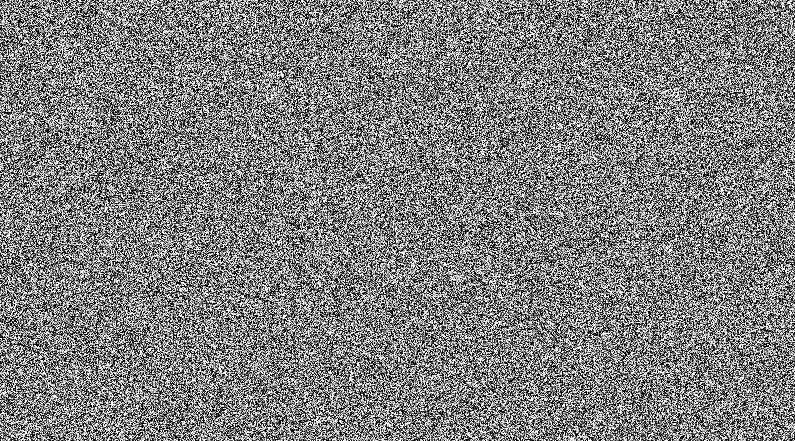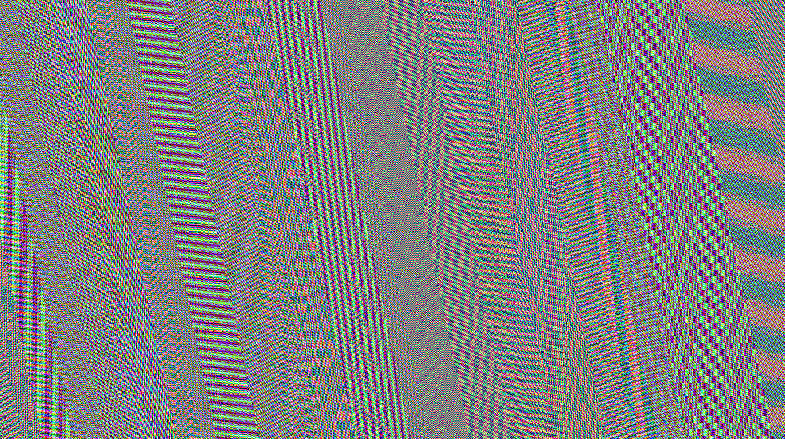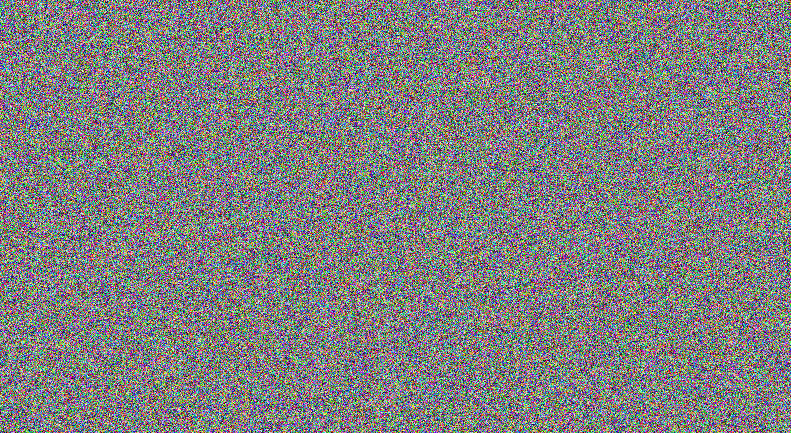## TL;DR

Random numbers are quite hard to generate, especially in shaders.

One interesting challenge I had to address whilst developing heist was how to get high quality good looking randomness into my shaders. For one particular effect I was working on I wanted a slight fuzz applied to the entire screen and this needed some randomness.

## Just Use A Texture Lookup

The obvious way to get randomness on the GPU is to generate it on the CPU. It’s really easy to generate high quality random numbers of the CPU and you can just stuff them into a texture and pass them to the GPU. You even generate yourself a random texture at compile time and just pass that in, great! Except… I wanted animated fuzz so I needed a different random number for every pixel every frame. Obviously you can extend the texture concept into a volume texture entirely full of random numbers and then move through the volume texture with time but that’s actually a pretty huge texture; 1920x1080x60 and that’s if you don’t mind repeating your randomness every second!

If this appeals to you, it would look something like this:

``````Sample NoiseVolume;
float Time;

{
float randomness = tex3D(NoiseVolume, float3(uv, Time)).r;
}
``````

#### Why Not Just Use A Smaller Texture?

I could just use a smaller texture and then wrap around my texture coordinates, thus using the same random values on different bits of the screen. The problem is the human eye is really good at spotting patterns, if there is any repetition in the randomess at all it will jump right out at you (because it’s the only pattern in there it sticks out like a sore thumb).

## Generating Random Numbers

Ok, so how hard can it be just to generate some random numbers? We just need a function a bit like this available to our pixel shader:

``````float Random(float2 uv, float seed);
``````

This should take those three floating point numbers and mix them up into a third totally unrelated one. Of course the usage of this shader is quite easy to implement when you have texture coordinates; just pass in the texture coordinates as uv and pass in some value, for example game time, as the seed). I went off to ShaderToy to experiment! ShaderToy is GLSL, but I can port it to HLSL once I’m done.My first though was that if I just multiply the numbers together and take the fractional part, that might be pretty random:

``````float Random_1(vec2 uv, float seed)
{
return fract(uv.x * uv.y * seed);
}
``````

As you can see it’s messy but it’s not really random. Not surprising for such a stupid random number generator. Another problem here is that the pattern starts off simple and gets more complex over time. I decided to fix that first since otherwise it would probably break all my future attempts after enough time had passed, which is a pain in the ass to debug.

I tried a variety of things to fix the seed into a small range.

Mod with a prime and then normalize:

``````float fixedSeed = mod(seed, 7621.0) / 7621.0 + 1.0;
``````

Divide by a prime and then take the fractional part:

``````float fixedSeed = fract(seed / 491.0) + 1.0;
``````

Just take the fractional part:

``````float fixedSeed = fract(seed) + 1.0;
``````

None of these really produced the results I was looking for. The last one being the worst because it repeats every second! I did realise, whilst experimenting with seeds that there was one serious problem - the seed being near zero often broke everything. That’s why all the examples above add 1.0 to the output, the seed is never near zero then. In the end I settled for making sure the seed is not near zero and putting up with testing the generator over a wide range of seeds.

I moved on to where all programmers go when not in their area of expertise: Stackoverflow. This answer recommended this for generating pixel randomness:``````float random( vec2 p )
{
// We need irrationals for pseudo randomness.
// Most (all?) known transcendental numbers will (generally) work.
const vec2 r = vec2(
23.1406926327792690,  // e^pi (Gelfond's constant)
2.6651441426902251); // 2^sqrt(2) (Gelfond–Schneider constant)
return fract( cos( mod( 123456789., 1e-7 + 256. * dot(p,r) ) ) );
}
``````

This does look pretty good! The only problem is it doesn’t use the seed and so it’s constant over time. My first instinct was just to try to multiply the seed in right at the end:

``````return fract(fixedSeed * cos( mod( 123456789., 1e-7 + 256. * dot(p,r) ) ) );
``````

Which sort of worked, but looked very weird over time. It felt like there was some temporal consistency. The particularly clever bit I liked about this generator was using the dot product with some irrational numbers - the problem my first generator had was that it had clear patterns varying over space. A dot product with irrational numbers removes the spatial component and gets us a nice, fairly random, floating point number. I tried multiplying the seed into the irrational vector:``````float fixedSeed = abs(seed) + 1.0;

//Mix our seed into the irrational vector
vec2 r = vec2(23.1406926327792690, 2.6651441426902251) * fixedSeed;

//Dot product with the irrationals (add 1 to ensure uv is not near zero)
float x = dot(uv + vec2(1, 1), r);
return fract( cos( mod( 123456789.0, 1e-7 + 256.0 * x ) ) );
``````

This produced pretty bad noise, with strange patterns flicking around. Before giving up on this line of enquiry I thought I’d try something else with x, something a little less complex than the StackOverflow method. Something like…

``````return fract(x);
``````

Not surprisingly, that didn’t quite work. It produced a complicated but regular pattern. Stealing another part from the StockOverflow solution I threw some trigonometry in there:

``````return fract(sin(x));
``````

This mixed it up a little more, but not quite enough. I thought maybe I should take some even less significant digits from the number, for example:

``````return fract(sin(x) * 100.0);
``````

This actually looked a lot better, there were still patterns and regularity but they were now a lot less obvious. Continuing with this trend I threw a really massive number in there and it looked pretty good:After a lot more fiddling, my final shader looks like this:

``````float Random_Final(vec2 uv, float seed)
{
float fixedSeed = abs(seed) + 1.0;
float x = dot(uv, vec2(12.9898,78.233) * fixedSeed);
return fract(sin(x) * 43758.5453);
}
``````

This has been tested with a wide range of UV values (far beyond what you should be putting in) as well as a huge range of time values (left running most of the day in the background).

## Is This Useful?

Your GPU could be bottlenecked in a few places (which are relevant to this discussion):

• Total Memory
• Texture Lookups (Memory bandwidth)
• Arithmetic

Using a lookup texture would cost you memory and bandwidth but save you arithmetic. Modern GPUs are pretty good at arithmetic but tend to be heavily loaded in terms of memory usage, which means saving yourself some memory space/bandwidth at the cost of a few ALU instructions is probably worth it.

## Why Aren’t You Using [Insert Well Known PRNG here]?

Random number generation is hardly a new topic! There are endless varieties of random number generators for every possible level of randomness quality, so why am I inventing my own? Almost every random number generator that I could find uses some bitwise operations (which are not available in the HLSL version I am targeting) or worked with integer operations which are slow on all but the most modern GPU architectures. This PRNG uses entirely floating point operations and should be very fast on all levels of GPU hardware.